TRIG.MA Measurement of Angles: Triangles, The Unit Circle, and Radian Measure
(i) Acute Angles.
For acute angles (in right triangles) $\Theta$ that are measured with degrees between 0 and 90 degrees, the sine and cosine are very useful and important trigonometric functions.
Also, for acute right triangles the sine and cosine are most easily measured when the hypotenuse of the right triangle has a unit measure, so $c= 1$.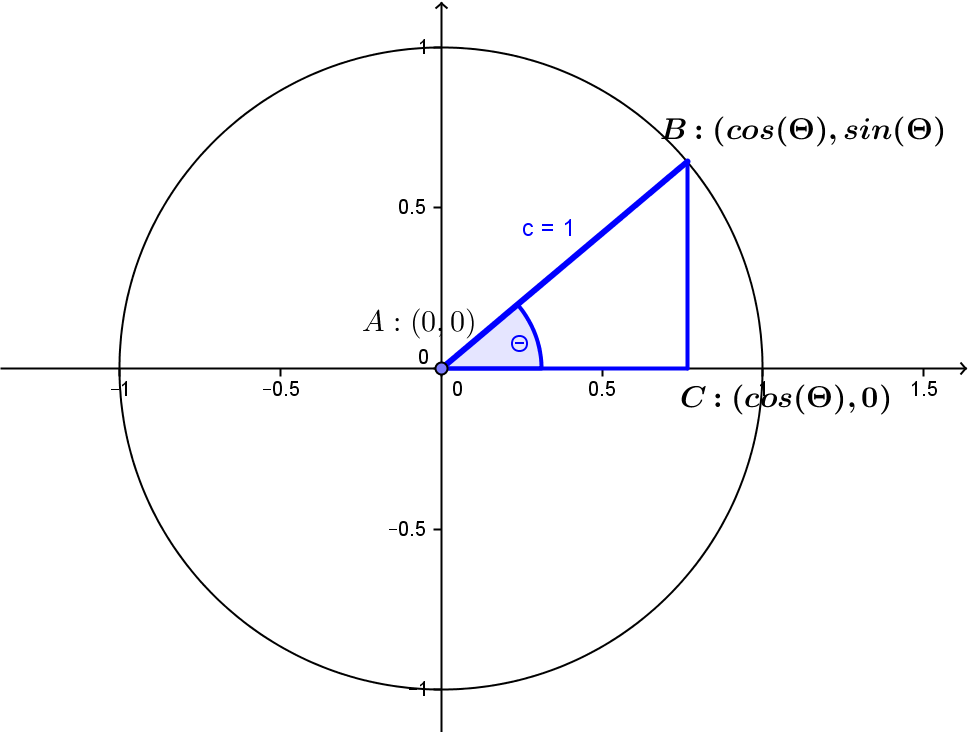These unit hypotenuse right triangles can all be considered in a cartesian plane with the vertex $A$ located with cartesian coordinates $(0,0)$,
$C$  with coordinates $(\cos(\Theta),0)$  and the vertex $B$ with coordinates $(\cos(\Theta),\sin(\Theta))$ on the unit circle with equation $x^2 + y^2 = 1$.

(ii) General triangles and interior angles that measure between 0 and 180 degrees.
In any triangle, the sum of the interior angles measures a straight angle ( 180 degrees).  If all the interior angles are acute, the triangle is called an acute triangle. If one of the angles measures 90 degrees, a right angle, the triangle is called a right triangle. If one of the angles measures more than 90 degrees, that angle is obtuse, and the triangle is called an obtuse triangle.

The general problem of triangles is to determine the measure of the three line segments and the three interior angles of a given triangle.
Based on the theory of similar triangles, trigonometry allows one to solve the general triangle problem from incomplete information using the sine and cosine functions for angles measured between 0 and 180 degrees.

See for more on solving triangles and vector geometry.

(iii) Circle Similarity and $\pi$:
For two circles $O, O'$ with circumferences $C, C'$; arcs cut by a central angle $a, a'$; diameters $d,d'$; and radii  $r,r'$: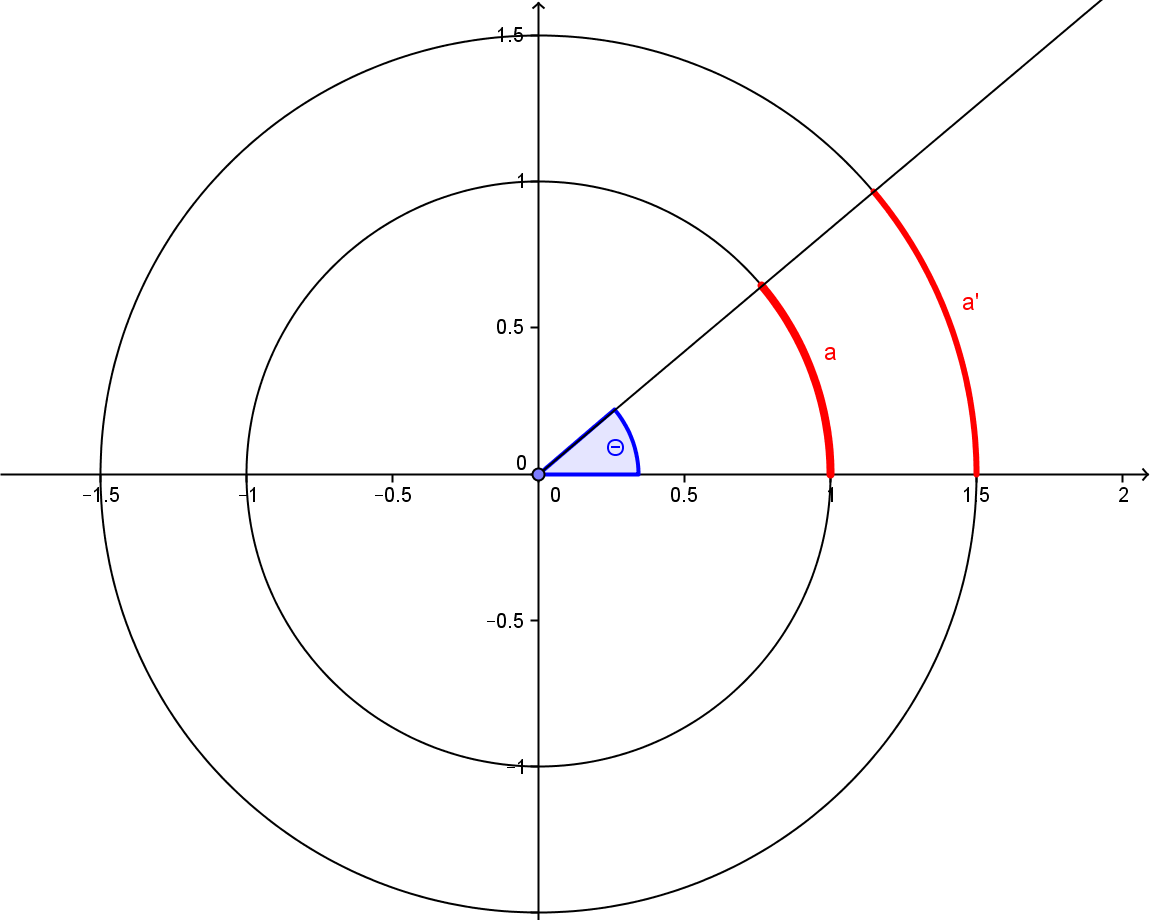$\frac {C'}C= \frac {d'}d=\frac {r'}r = \frac {a'}a$.
So $C/d =C'/d'$ is a constant (well known), denoted by the Greek letter,$\pi$.
$\pi$ is an irrational number. In fact, $\pi$ is a transcendental number, meaning that there is no polynomial function,$p$ with integer coefficients where $p(\pi) = 0$. These two facts are not simple to prove.
Result: $C = \pi*d = 2 \pi * r$

(iv) Central Circular Angles and Radian Measure
Central angles in a (unit) circle cut the circle in arcs.
The ratio of the arc lengths $t, t'$ is proportional to the ratio of the corresponding angle measurements $\Theta , \Theta'$  (in degrees).
$\Theta' /\Theta= t' /t$ or $\Theta/t = \Theta'/t'$.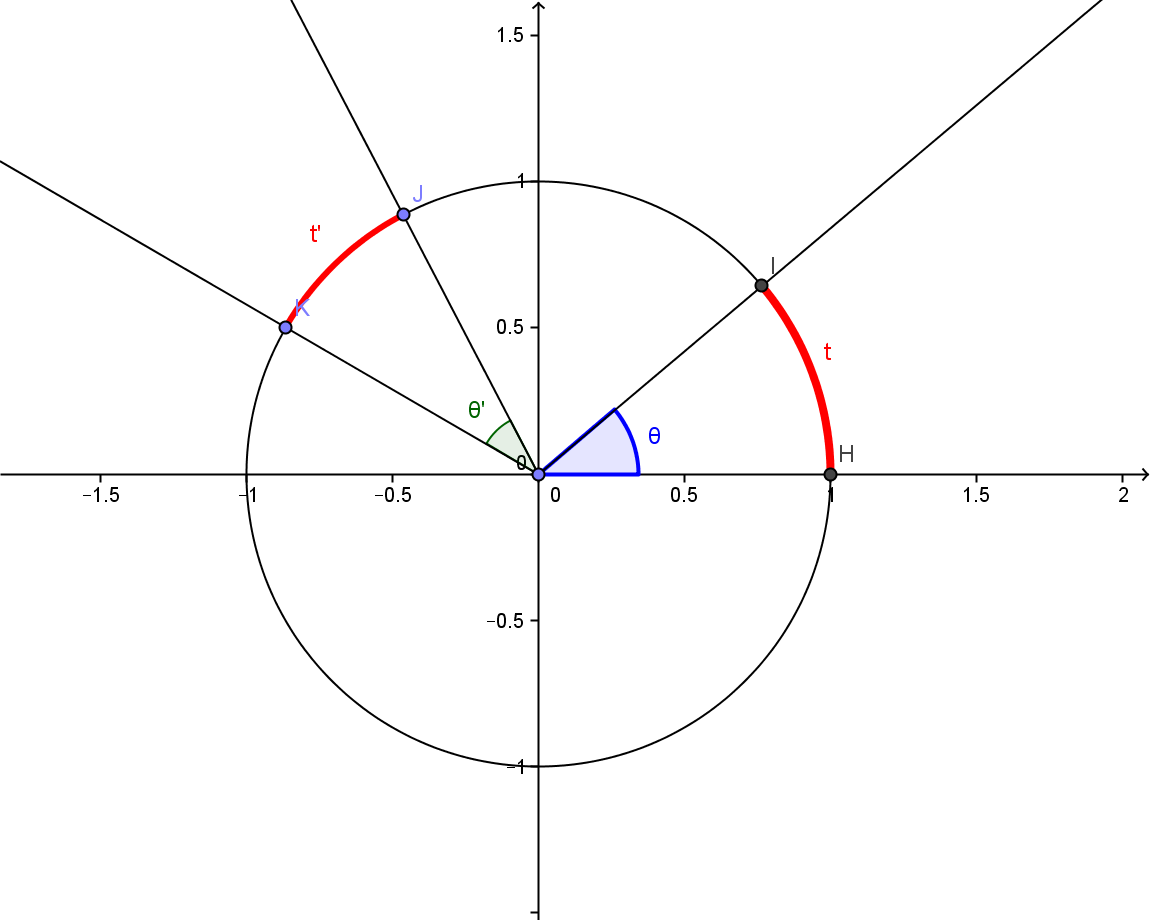In a unit circle, the arc length determined by a central angle is  called the radian measure of the angle. One radian measures the central angle that cuts an arc of length 1 on a circle with radius 1.
Also, $\pi$  radians measures a central angle of  $180$  degrees, a straight angle, and $\pi /2$ radians  measures a central angle of $90$ degrees, a right angle.
It is customary to use the variable "$t$" to denote the measure of an angle with radian measure.
$\Theta/180 = t /π$ where  $\Theta$ is the measure of  an angle in degrees and  $t$ is the measure of the same angle in radians.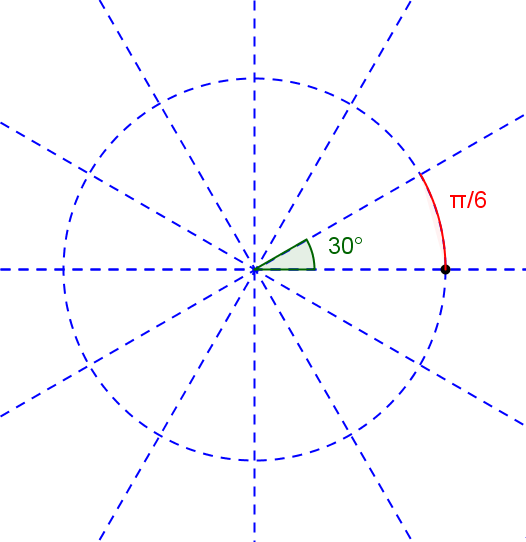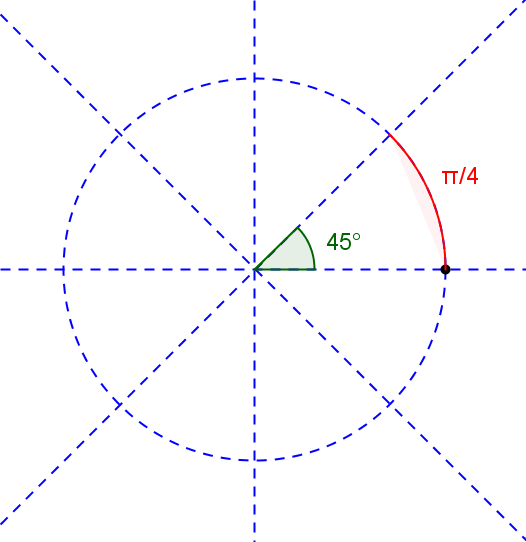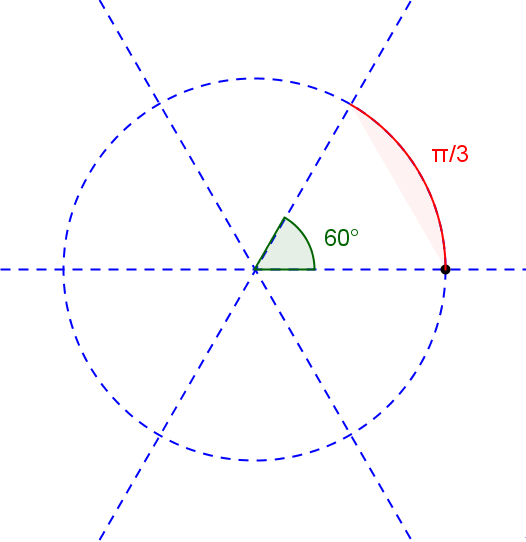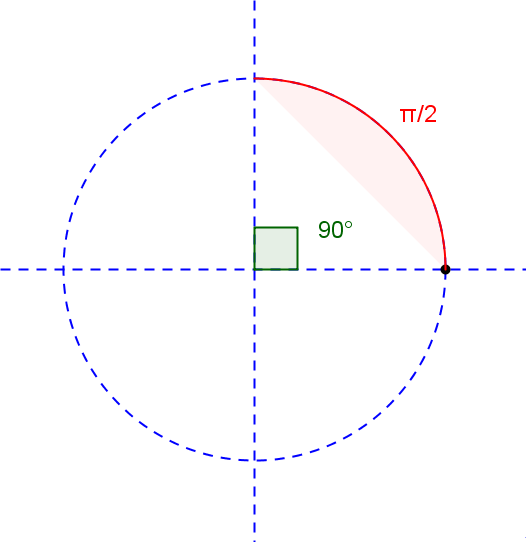Entries in the table below show the corresponding measurements of some common angles with measure $\Theta$ degrees and measure $t$ radians. The adjacent mapping diagram visualizes the scale correspondence between degree and radian measures of an angle.

 $\Theta$ t 360 $2\pi$ 270 $3\pi/2$ 180 $\pi$ 90 $\pi/2$ 0 0 60 $\pi/3$ 45 $\pi/4$ 30 $\pi/6$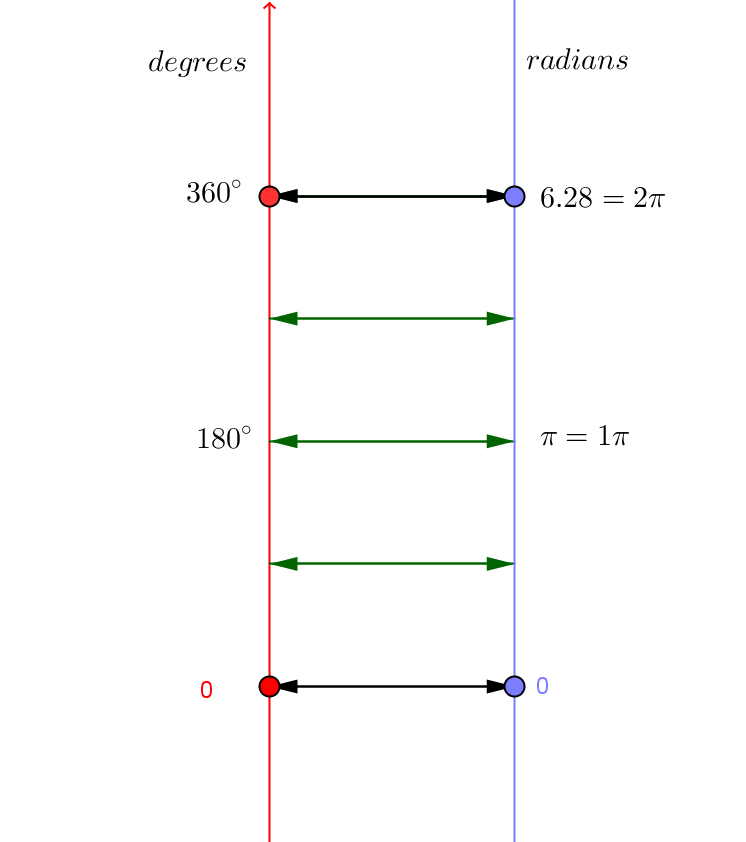In the following GeoGebra mapping diagram you can control the angle using the x mark (in orange) on the $degrees$ axis which is initially set at $45$ degrees. This will change the central angle and corresponding arc on the unit circle as well as indicate the corresponding measure in radians for the angle.